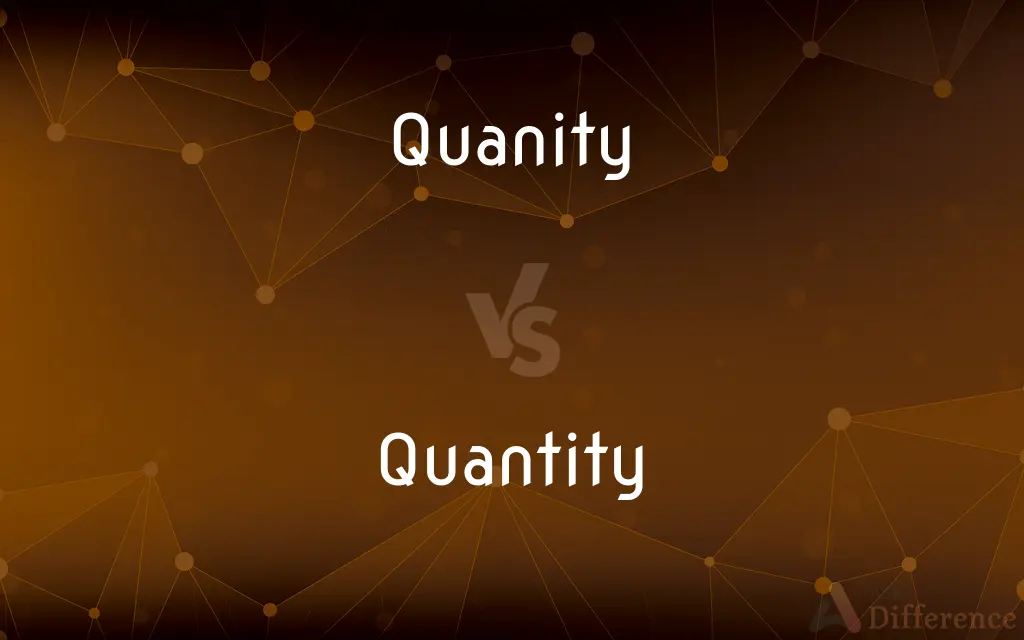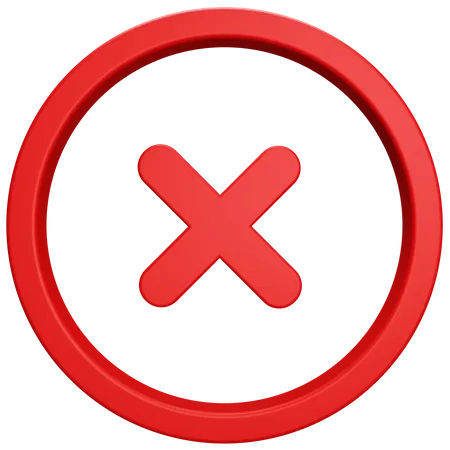# Quanity vs. Quantity — What's the Difference?

"Quanity" is a misspelling; "Quantity" refers to an amount or number of something.## Which is correct: Quanity or Quantity

How to spell Quantity?### Quanity

Incorrect SpellingCorrect Spelling

## Key Differences

Recall "quantity" has the same "tity" ending as "sanctity."
Think of "quantum" when spelling "quantity."
Ensure you have both the "t" and "i" before the "ty" ending.
Remember, there's no word as "quanity" in the dictionary.
Associate "quantity" with "quality" – both have a "qu" beginning and similar structures.

## Compare with Definitions

#### Quanity

Quanity is an incorrect spelling of Quantity.

#### Quantity

A unit or grade of measure.
They ordered a larger quantity of bricks for the project.

#### Quantity

The property of something related to its magnitude.
The quantity of work this week is overwhelming.

#### Quantity

A certain, often considerable, amount.
The quantity of water in the ocean is vast.

#### Quantity

A specified or indefinite number or amount
Shipped a large quantity of books.
Sells quantities of paper to publishers.

#### Quantity

A considerable amount or number
Sells drugs wholesale and in quantity.

#### Quantity

An exact amount or number
The quantity of material recycled in a month.

#### Quantity

The measurable or countable property or aspect of things
Arithmetic deals with quantity.

#### Quantity

(Mathematics) Something that serves as the object of an operation.

#### Quantity

(Linguistics) The relative amount of time needed to pronounce a vowel, consonant, or syllable.

#### Quantity

The duration of a syllable in quantitative verse.

#### Quantity

(Logic) The exact character of a proposition in reference to its universality, singularity, or particularity.

#### Quantity

A fundamental, generic term used when referring to the measurement (count, amount) of a scalar, vector, number of items or to some other way of denominating the value of a collection or group of items.
You have to choose between quantity and quality.

#### Quantity

An indefinite amount of something.
Some soap making oils are best as base oils, used in a larger quantity in the soap, while other oils are best added in a small quantity.
Olive oil can be used practically in any quantity.

#### Quantity

A specific measured amount.
This bag would normally costs \$497.50 for a quantity of 250, at a price of \$1.99 per piece.
Generally it should not be used in a quantity larger than 15 percent.

#### Quantity

A considerable measure or amount.
The Boeing P-26A was the first all-metal monoplane fighter produced in quantity for the U.S. Army Air Corps.

#### Quantity

(metrology) Property of a phenomenon, body, or substance, where the property has a magnitude that can be expressed as number and a reference.

#### Quantity

(mathematics) Indicates that the entire preceding expression is henceforth considered a single object.
X plus y quantity squared equals x squared plus 2xy plus y squared.

#### Quantity

(phonology) Length of sounds.

#### Quantity

The attribute of being so much, and not more or less; the property of being measurable, or capable of increase and decrease, multiplication and division; greatness; and more concretely, that which answers the question "How much?"; measure in regard to bulk or amount; determinate or comparative dimensions; measure; amount; bulk; extent; size.

#### Quantity

That which can be increased, diminished, or measured; especially (Math.), anything to which mathematical processes are applicable.

#### Quantity

A determinate or estimated amount; a sum or bulk; a certain portion or part; sometimes, a considerable amount; a large portion, bulk, or sum; as, a medicine taken in quantities, that is, in large quantities.
The quantity of extensive and curious information which he had picked up during many months of desultory, but not unprofitable, study.

#### Quantity

How much there is of something that you can quantify

#### Quantity

He had a quantity of ammunition

#### Quantity

Something that has a magnitude and can be represented in mathematical expressions by a constant or a variable

#### Quantity

The amount or number of a material or immaterial thing.
I was amazed by the quantity of books in the library.

#### Quantity

A measurable aspect; a specific portion.
The recipe requires a small quantity of sugar.

## Common Curiosities

#### Why is it called Quantity?

It's called "quantity" from Latin "quantitas" meaning "how much."

"Quantity."

#### What is the verb form of Quantity?

There isn't a direct verb form for "quantity." However, "quantify" is related.

#### What is the pronunciation of Quantity?

The pronunciation is /ˈkwɑːn.tə.ti/.

"Quantities."

#### What is the root word of Quantity?

The root is derived from Latin "quantitas."

#### Which vowel is used before Quantity?

"A" as in "a quantity."

#### Which preposition is used with Quantity?

"Of" as in "quantity of items."

#### Which conjunction is used with Quantity?

Any conjunction can be used depending on the sentence, like "and" or "but."

No, "quantity" is not an adverb.

#### Is Quantity an abstract noun?

No, it's a concrete noun when referring to specific amounts but can be abstract when referring to general magnitude.

#### Is Quantity a countable noun?

Yes, you can have quantities (plural) of something.

It's neutral.

Quan-ti-ty.

#### What is a stressed syllable in Quantity?

The first syllable "Quan" is stressed.

#### Which determiner is used with Quantity?

"Such" as in "such quantity."

#### Which article is used with Quantity?

Either "a" or "the" depending on the context.

#### Is the Quantity term a metaphor?

Not inherently, but it can be used metaphorically.

Three syllables.

#### What is the opposite of Quantity?

"Quality" when contrasting aspects.

#### Is Quantity a noun or adjective?

"Quantity" is a noun.

No, it isn't.

#### Is the word Quantity imperative?

No, "quantity" is a noun, not a verb.

#### Is Quantity a vowel or consonant?

"Quantity" is a word, not a letter, so it's neither a vowel nor consonant.

#### What part of speech is Quantity?

"Quantity" is a noun.

"Amount."

#### What is the first form of Quantity?

"Quantity" as it's a noun and doesn't have verb forms.

#### What is the second form of Quantity?

Not applicable as "quantity" is a noun.

#### What is the third form of Quantity?

Not applicable as "quantity" is a noun.

#### How is Quantity used in a sentence?

"We need to determine the quantity of supplies required for the project."